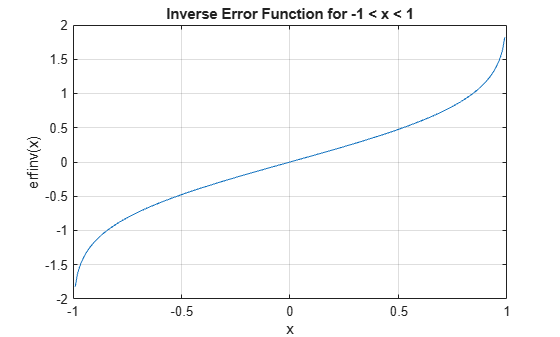erfinv

Inverse error function

Description

example

erfinv(x) returns the Inverse Error Function evaluated for each element of x. For inputs outside the interval [-1 1], erfinv returns NaN.

Examples

collapse all

erfinv(0.25)
ans = 0.2253

For inputs outside [-1,1], erfinv returns NaN. For -1 and 1, erfinv returns -Inf and Inf, respectively.

erfinv([-2 -1 1 2])
ans = 1×4

NaN  -Inf   Inf   NaN

Find the inverse error function of the elements of a matrix.

M = [0 -0.5; 0.9 -0.2];
erfinv(M)
ans = 2×2

0   -0.4769
1.1631   -0.1791

Plot the inverse error function for -1 < x < 1.

x = -1:0.01:1;
y = erfinv(x);
plot(x,y)
grid on
xlabel('x')
ylabel('erfinv(x)')
title('Inverse Error Function for -1 < x < 1')Generate Gaussian distributed random numbers using uniformly distributed random numbers. To convert a uniformly distributed random number $x$ to a Gaussian distributed random number $y$, use the transform

$y=\sqrt{2}er{f}^{-1}\left(x\right).$

Note that because x has the form -1 + 2*rand(1,10000), you can improve accuracy by using erfcinv instead of erfinv. For details, see Tips.

Generate 10,000 uniformly distributed random numbers on the interval [-1,1]. Transform them into Gaussian distributed random numbers. Show that the numbers follow the form of the Gaussian distribution using a histogram plot.

rng('default')
x = -1 + 2*rand(1,10000);
y = sqrt(2)*erfinv(x);
h = histogram(y);Input Arguments

collapse all

Input, specified as a real number, or a vector, matrix, or multidimensional array of real numbers. x cannot be sparse.

Data Types: single | double

collapse all

Inverse Error Function

The inverse error function erfinv is defined as the inverse of the error function, such that

$\text{erfinv}\left(\text{erf}\left(x\right)\right)=x.$

Tips

• For expressions of the form erfinv(1-x), use the complementary inverse error function erfcinv instead. This substitution maintains accuracy. When x is close to 1, then 1 - x is a small number and may be rounded down to 0. Instead, replace erfinv(1-x) with erfcinv(x).